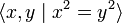# Amalgamated free product of Z and Z over 2Z

View a complete list of particular groups (this is a very huge list!)[SHOW MORE]

## Definition

### Definition as an amalgamated free product

The group is defined as the amalgamated free product$\mathbb{Z} *_{2\mathbb{Z}} \mathbb{Z}$, i.e., we take two copies of the group of integers, take their free product, and then take the quotient group by the identification of the subgroup$2\mathbb{Z}$.

### Definition by presentation

The group can be defined by the presentation:$\langle x,y \mid x^2 = y^2 \rangle$

## Group properties

Property Meaning Satisfied? Explanation
abelian group any two elements commute No$x,y$ do not commute
nilpotent group upper central series reaches the whole group in finitely many steps No The quotient group by the center$\langle x^2 \rangle$ is isomorphic to the infinite dihedral group, which is centerless.
solvable group has a normal series where all the quotients are abelian Yes The quotient by the center is infinite dihedral group, which is a metacyclic group.